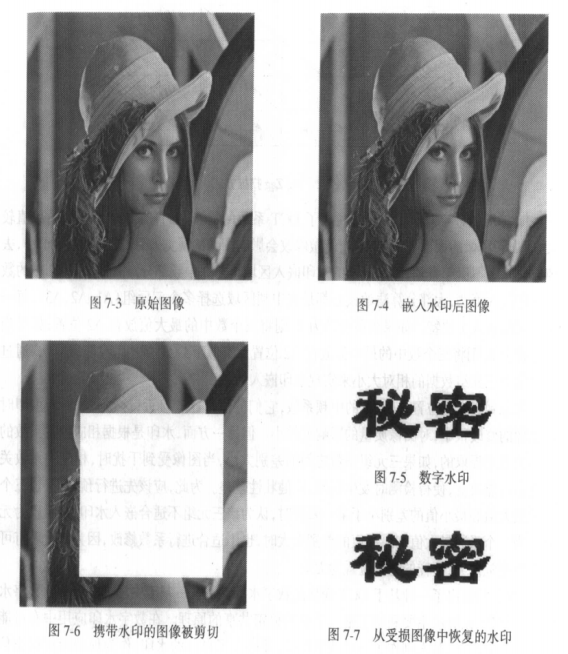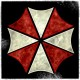• 高考得分神器！edu.gkroot.com|>>>>>>>>>><<<<<<<<<<( 2015-12-15 15:48:53 )

## 阿里巴巴公司根据截图查到泄露信息的具体员工的技术是什么？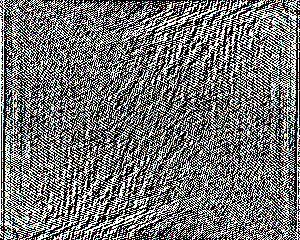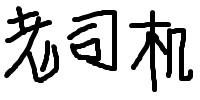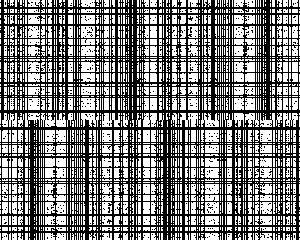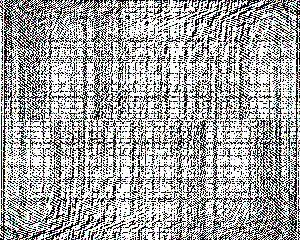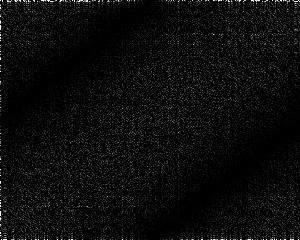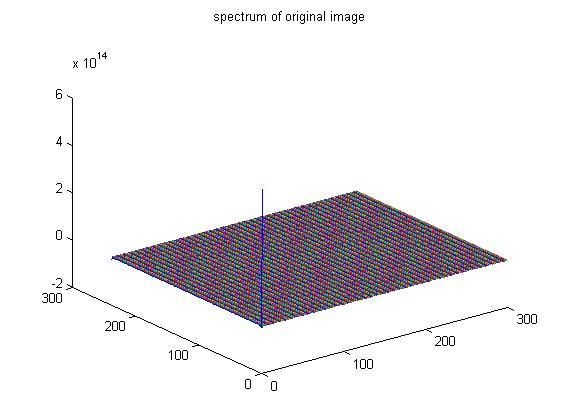水印提取是水印叠加的逆过程，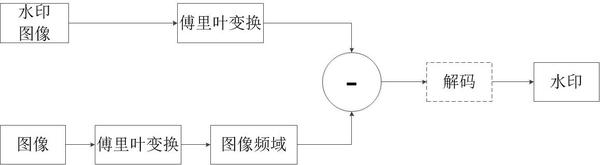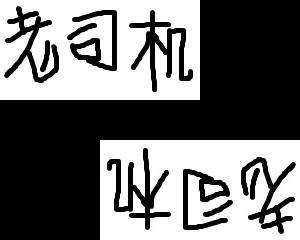1.进行涂抹攻击，这是攻击后的图片：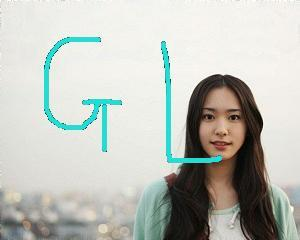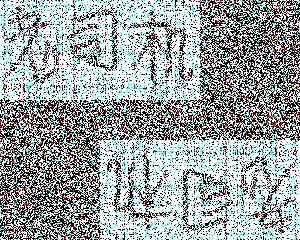2.进行剪切攻击，就是网上经常用的截图截取一部分的情况：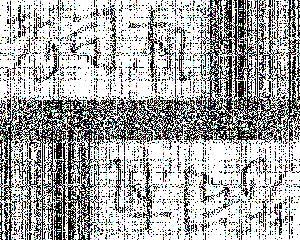3.伸缩攻击（这个实验明码做的，水印能量较高，隐匿性不强）：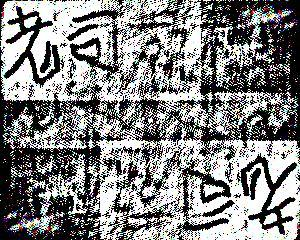4.旋转攻击（明码）：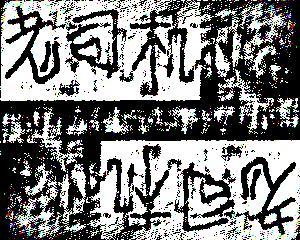5.JPEG压缩后（这个实验我好像是拿明码做的，能量主要加在了高频）：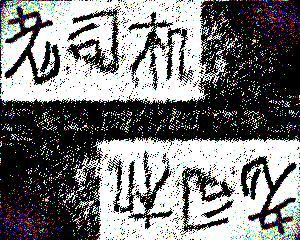6.PS 4像素马赛克/均值滤波等，攻击后图像(这是我女朋友吗？丑死了)：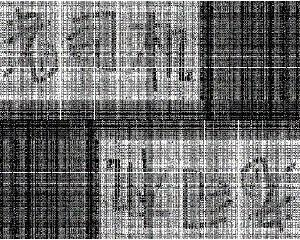7.截屏，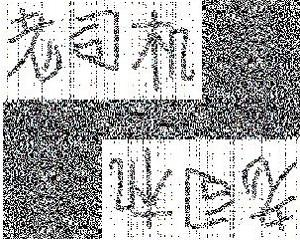8. 亮度调节（明码）：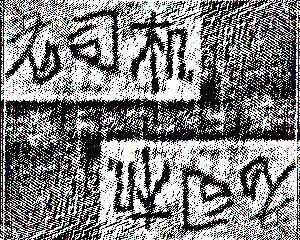9.色相调节（明码）：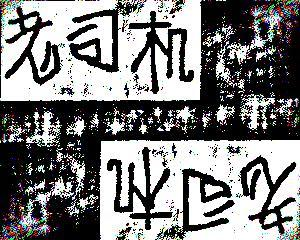10.饱和度调节（明码）：11.对比度（明码）：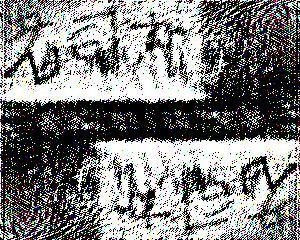12.评论区用waifu2x去噪后图片：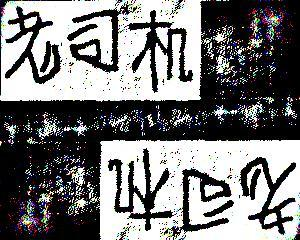13.美图秀秀，我对我女票一键美颜，美白，磨皮，加腮红，加唇彩（有一种很羞耻的感觉，捂脸）：14.对于背景纯色的图其实也是无所谓的能量系数为10时加水印图片：觉得太显噪就把能量系数调低，不过水印的隐秘性和鲁棒性是互斥的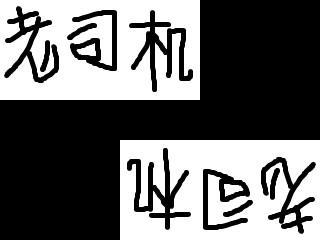15.我用将RGB>600的像素设置成为(0，255，0)来模拟PS魔术手，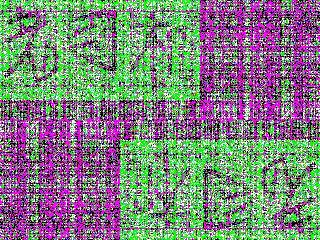16.屏摄，好吧，这个实验我做哭了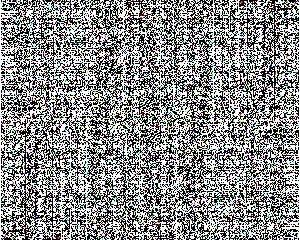=====彩蛋====@杨一丁 在答案中给出了上图隐写的内容，（雾）。

******************************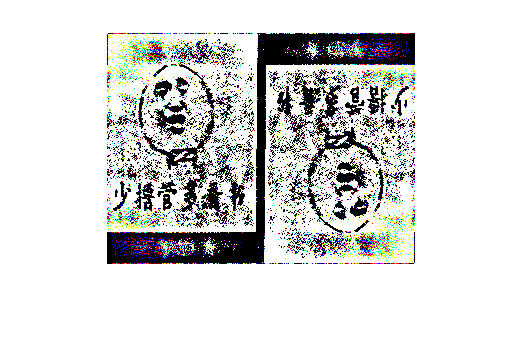```%%傅里叶变换加水印源代码
%% 运行环境Matlab2010a
clc;clear;close all;
alpha = 1;
figure, imshow(im),title('original image');
figure, imshow(mark),title('watermark');
%% encode mark
imsize = size(im);
%random
TH=zeros(imsize(1)*0.5,imsize(2),imsize(3));
TH1 = TH;
TH1(1:size(mark,1),1:size(mark,2),:) = mark;
M=randperm(0.5*imsize(1));
N=randperm(imsize(2));
save('encode.mat','M','N');
for i=1:imsize(1)*0.5
for j=1:imsize(2)
TH(i,j,:)=TH1(M(i),N(j),:);
end
end
% symmetric
mark_ = zeros(imsize(1),imsize(2),imsize(3));
mark_(1:imsize(1)*0.5,1:imsize(2),:)=TH;
for i=1:imsize(1)*0.5
for j=1:imsize(2)
mark_(imsize(1)+1-i,imsize(2)+1-j,:)=TH(i,j,:);
end
end
figure,imshow(mark_),title('encoded watermark');
%imwrite(mark_,'encoded watermark.jpg');
FA=fft2(im);
figure,imshow(FA);title('spectrum of original image');
FB=FA+alpha*double(mark_);
figure,imshow(FB); title('spectrum of watermarked image');
FAO=ifft2(FB);
figure,imshow(FAO); title('watermarked image');
%imwrite(uint8(FAO),'watermarked image.jpg');
RI = FAO-double(im);
figure,imshow(uint8(RI)); title('residual');
%imwrite(uint8(RI),'residual.jpg');
xl = 1:imsize(2);
yl = 1:imsize(1);
[xx,yy] = meshgrid(xl,yl);
figure, plot3(xx,yy,FA(:,:,1).^2+FA(:,:,2).^2+FA(:,:,3).^2),title('spectrum of original image');
figure, plot3(xx,yy,FB(:,:,1).^2+FB(:,:,2).^2+FB(:,:,3).^2),title('spectrum of watermarked image');
figure, plot3(xx,yy,FB(:,:,1).^2+FB(:,:,2).^2+FB(:,:,3).^2-FA(:,:,1).^2+FA(:,:,2).^2+FA(:,:,3).^2),title('spectrum of watermark');
%% extract watermark
FA2=fft2(FAO);
G=(FA2-FA)/alpha;
GG=G;
for i=1:imsize(1)*0.5
for j=1:imsize(2)
GG(M(i),N(j),:)=G(i,j,:);
end
end
for i=1:imsize(1)*0.5
for j=1:imsize(2)
GG(imsize(1)+1-i,imsize(2)+1-j,:)=GG(i,j,:);
end
end
figure,imshow(GG);title('extracted watermark');
%imwrite(uint8(GG),'extracted watermark.jpg');
%% MSE and PSNR
C=double(im);
RC=double(FAO);
MSE=0; PSNR=0;
for i=1:imsize(1)
for j=1:imsize(2)
MSE=MSE+(C(i,j)-RC(i,j)).^2;
end
end
MSE=MSE/360.^2;
PSNR=20*log10(255/sqrt(MSE));
MSE
PSNR

%% attack test
%% attack by smearing
figure,imshow(attack);
FAO_ = FAO;
for i=1:imsize(1)
for j=1:imsize(2)
if attack(i,j,1)+attack(i,j,2)+attack(i,j,3)>0.5
FAO_(i,j,:) = attack(i,j,:);
end
end
end
figure,imshow(FAO_);
%extract watermark
FA2=fft2(FAO_);
G=(FA2-FA)*2;
GG=G;
for i=1:imsize(1)*0.5
for j=1:imsize(2)
GG(M(i),N(j),:)=G(i,j,:);
end
end
for i=1:imsize(1)*0.5
for j=1:imsize(2)
GG(imsize(1)+1-i,imsize(2)+1-j,:)=GG(i,j,:);
end
end
figure,imshow(GG);title('extracted watermark');

%% attack by cutting
s2 = 0.8;
FAO_ = FAO;
FAO_(:,s2*imsize(2)+1:imsize(2),:) = FAO_(:,1:int32((1-s2)*imsize(2)),:);
figure,imshow(FAO_);
%extract watermark
FA2=fft2(FAO_);
G=(FA2-FA)*2;
GG=G;
for i=1:imsize(1)*0.5
for j=1:imsize(2)
GG(M(i),N(j),:)=G(i,j,:);
end
end
for i=1:imsize(1)*0.5
for j=1:imsize(2)
GG(imsize(1)+1-i,imsize(2)+1-j,:)=GG(i,j,:);
end
end
figure,imshow(GG);title('extracted watermark');

%%小波变换加水印，解水印大家按照加的思路逆过来就好
clc;clear;close all;
figure, imshow(im),title('original image');
figure, imshow(mark),title('watermark');
%% RGB division
im=double(im);
mark=double(mark);
imr=im(:,:,1);
markr=mark(:,:,1);
img=im(:,:,2);
markg=mark(:,:,2);
imb=im(:,:,3);
markb=mark(:,:,3);
%% parameter
r=0.04;
g = 0.04;
b = 0.04;
%% wavelet tranform and add watermark
% for red
[Cwr,Swr]=wavedec2(markr,1,'haar');
[Cr,Sr]=wavedec2(imr,2,'haar');
Cr(1:size(Cwr,2)/16)=...
Cr(1:size(Cwr,2)/16)+r*Cwr(1:size(Cwr,2)/16);
k=0;
while k<=size(Cr,2)/size(Cwr,2)-1
Cr(1+size(Cr,2)/4+k*size(Cwr,2)/4:size(Cr,2)/4+...
(k+1)*size(Cwr,2)/4)=Cr(1+size(Cr,2)/4+...
k*size(Cwr,2)/4:size(Cr,2)/4+(k+1)*size(Cwr,2)/4)+...
r*Cwr(1+size(Cwr,2)/4:size(Cwr,2)/2);
Cr(1+size(Cr,2)/2+k*size(Cwr,2)/4:size(Cr,2)/2+...
(k+1)*size(Cwr,2)/4)=Cr(1+size(Cr,2)/2+...
k*size(Cwr,2)/4:size(Cr,2)/2+(k+1)*size(Cwr,2)/4)+...
r*Cwr(1+size(Cwr,2)/2:3*size(Cwr,2)/4);
Cr(1+3*size(Cwr,2)/4+k*size(Cwr,2)/4:3*size(Cwr,2)/4+...
(k+1)*size(Cwr,2)/4)=Cr(1+3*size(Cr,2)/4+...
k*size(Cwr,2)/4:3*size(Cr,2)/4+(k+1)*size(Cwr,2)/4)+...
r*Cwr(1+3*size(Cwr,2)/4:size(Cwr,2));
k=k+1;
end;
Cr(1:size(Cwr,2)/4)=Cr(1:size(Cwr,2)/4)+r*Cwr(1:size(Cwr,2)/4);

% for green
[Cwg,Swg]=WAVEDEC2(markg,1,'haar');
[Cg,Sg]=WAVEDEC2(img,2,'haar');
Cg(1:size(Cwg,2)/16)=...
Cg(1:size(Cwg,2)/16)+g*Cwg(1:size(Cwg,2)/16);
k=0;
while k<=size(Cg,2)/size(Cwg,2)-1
Cg(1+size(Cg,2)/4+k*size(Cwg,2)/4:size(Cg,2)/4+...
(k+1)*size(Cwg,2)/4)=Cg(1+size(Cg,2)/4+...
k*size(Cwg,2)/4:size(Cg,2)/4+(k+1)*size(Cwg,2)/4)+...
g*Cwg(1+size(Cwg,2)/4:size(Cwg,2)/2);
Cg(1+size(Cg,2)/2+k*size(Cwg,2)/4:size(Cg,2)/2+...
(k+1)*size(Cwg,2)/4)=Cg(1+size(Cg,2)/2+...
k*size(Cwg,2)/4:size(Cg,2)/2+(k+1)*size(Cwg,2)/4)+...
g*Cwg(1+size(Cwg,2)/2:3*size(Cwg,2)/4);
Cg(1+3*size(Cg,2)/4+k*size(Cwg,2)/4:3*size(Cg,2)/4+...
(k+1)*size(Cwg,2)/4)=Cg(1+3*size(Cg,2)/4+...
k*size(Cwg,2)/4:3*size(Cg,2)/4+(k+1)*size(Cwg,2)/4)+...
g*Cwg(1+3*size(Cwg,2)/4:size(Cwg,2));
k=k+1;
end;
Cg(1:size(Cwg,2)/4)=Cg(1:size(Cwg,2)/4)+g*Cwg(1:size(Cwg,2)/4);

% for blue
[Cwb,Swb]=WAVEDEC2(markb,1,'haar');
[Cb,Sb]=WAVEDEC2(imb,2,'haar');
Cb(1:size(Cwb,2)/16)+b*Cwb(1:size(Cwb,2)/16);
k=0;
while k<=size(Cb,2)/size(Cwb,2)-1
Cb(1+size(Cb,2)/4+k*size(Cwb,2)/4:size(Cb,2)/4+...
(k+1)*size(Cwb,2)/4)=Cb(1+size(Cb,2)/4+...
k*size(Cwb,2)/4:size(Cb,2)/4+(k+1)*size(Cwb,2)/4)+...
g*Cwb(1+size(Cwb,2)/4:size(Cwb,2)/2);
Cb(1+size(Cb,2)/2+k*size(Cwb,2)/4:size(Cb,2)/2+...
(k+1)*size(Cwb,2)/4)=Cb(1+size(Cb,2)/2+...
k*size(Cwb,2)/4:size(Cb,2)/2+(k+1)*size(Cwb,2)/4)+...
b*Cwb(1+size(Cwb,2)/2:3*size(Cwb,2)/4);
Cb(1+3*size(Cb,2)/4+k*size(Cwb,2)/4:3*size(Cb,2)/4+...
(k+1)*size(Cwb,2)/4)=Cb(1+3*size(Cb,2)/4+...
k*size(Cwb,2)/4:3*size(Cb,2)/4+(k+1)*size(Cwb,2)/4)+...
b*Cwb(1+3*size(Cwb,2)/4:size(Cwb,2));
k=k+1;
end;
Cb(1:size(Cwb,2)/4)=Cb(1:size(Cwb,2)/4)+b*Cwb(1:size(Cwb,2)/4);
%% image reconstruction
imr=WAVEREC2(Cr,Sr,'haar');
img=WAVEREC2(Cg,Sg,'haar');
imb=WAVEREC2(Cb,Sb,'haar');
imsize=size(imr);
FAO=zeros(imsize(1),imsize(2),3);
for i=1:imsize(1);
for j=1:imsize(2);
FAO(i,j,1)=imr(i,j);
FAO(i,j,2)=img(i,j);
FAO(i,j,3)=imb(i,j);
end
end
figure, imshow(FAO); title('watermarked image');```

### 他人回答：

1.重要的图片或者文档内加水印，文件里面再加上全息数字水印（这个大家都说得很全面了）

2.所有终端监控敏感操作：另存、截屏、剪贴板、对敏感文档的操作等等，上述行为一律上传到审计服务器上备份待查。

3.禁止未授权（未安装终端防护程序）的终端接入内网和业务系统。

4.有敏感操作或者跨终端登录的时候通过摄像头拍摄人脸（知道为什么扎克伯格要贴摄像头了吧）

5.定期对硬盘进行文件扫描，关键字、相似度、OCR等方法识别出可能是敏感或者涉密的文件，并提取特征，进行标定。

6.同样采用散列值、关键字、相似度匹配等方法检查所有网络出口：邮件、聊天、网站、网盘等，对传输内容全部解码进行审计并且记录。

7.检查或者关闭所有物理出口，USB、Wi-Fi、蓝牙、音频口（防止通过耳机口盗窃数据）。

8.所有文件落地加密（文件加密或者全盘加密），偷硬盘也没用。

9.所有电子设备进出办公区域全部审核检查。

10.核心机密文档不落地，存放于核心服务器，采用远程终端或者虚拟窗口方式查阅/编辑。